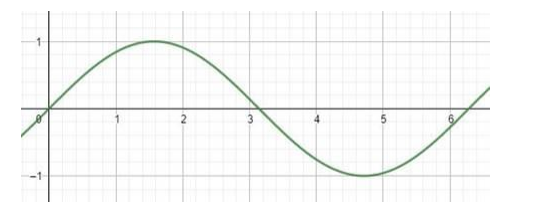# Find the range of the function f`
Question:

Find the range of the function f(x) = sin x.

Solution:

The graph of sin(x) isSin(x) is a periodic function whose values always lies between -1 to +1. The maximum

value is attained at $n^{\frac{\pi}{2}}$ where $n$ is odd and minimum when $n$ is even. Hence, Range is $[-1,+1]$.